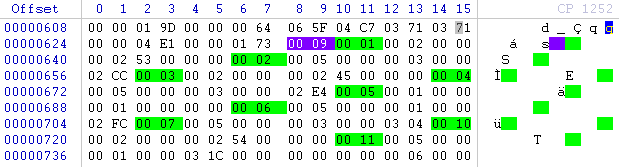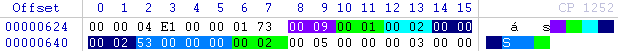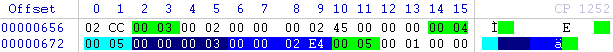## Monday, 4 March 2013

### Location Data within JPGs

We have become accustomed to fact that many of our digital photographs have location data embedded within them, populated with a GPS receiver. This data is often utilized by the modern photo management programs such as iPhoto and could conceivably have some evidential value at some point.  So where is it stored? You are probably thinking, like I was, that it was sat along with all that other Exif data, is possibly in plain text and that it would be easy to locate and retrieve.  In fact there is a little more to it.

In Figure 1 we can see the first part of a jpg taken with an iPhone5.

﻿﻿Figure 1
My readers will recognize the start of the jpg header 0xFF 0xD8 0xFF  etc etc but I want to draw your attention to 0x4D 0x4D .  These two bytes are the start of a section of data known as a TIFF structure and are part of an 8 byte header.  The TIFF file specification published by Adobe details the information residing in this header.

﻿Figure 2

In ASCII these bytes are read as MM.  These bytes indicate that where applicable the data following should be interpreted Big Endian.  The MM and II references hark back to the competing Intel and Motorola technologies.  Immediately following are the two bytes 0x00 0x2A which decoded as an (in this case big endian) integer have the value 42.  This value 42 always follows MM or II and is part of the TIFF structure header (note the HHGTTG reference). The following four bytes 00 00 00 08 detail the offset (8 in this case) from the start of the TIFF structure header to the start of a structure called an IFD.

IFD is an Image File Directory. Adobe defines it as: a 2 byte count of the number of directory entries (i.e. the number of fields), followed by a sequence of 12 byte field entries, followed by a 4 byte offset of the next IFD (or 0 if none).

We can see that in this case we have 0x00 0x0a (10) 12 byte directory entries the start of each being marked in green.  Adobe details the structure of each entry as follows:

Bytes 0-1 The tag that identifies the field

Bytes 2-3 The field type

Bytes 4-7 the number of values, Count of the indicated type

Bytes 8-11 The Value Offset, the file offset (in bytes) of the Value of the field

The tag we are interested in within the IFD is 0x88 0x25. A comprehensive list of tag numbers can be found at www.exiv2.org/tags.html. The 0x88 0x25 tag is used to store a pointer to another IFD, the GPS Info IFD. If this tag does not exist there isn't a GPS Info IFD and therefore no JPG location information (but don't forget the tag may be stored little endian 0x25 0x88). Bytes 2-3 in this directory entry are 0x00 0x04. This indicates that the field type is type 4. Adobe details the possible field types as follows:

1 = BYTE 8-bit unsigned integer.

2 = ASCII 8-bit byte that contains a 7-bit ASCII code; the last byte must be NUL (binary zero).

3 = SHORT 16-bit (2-byte) unsigned integer.

4 = LONG 32-bit (4-byte) unsigned integer.

5 = RATIONAL Two LONGs: the first represents the numerator of a fraction; the second, the denominator.

We can see that the field type is 4 indicating that the value of this entry will be stored as one or more (depending on the Count)  32 bit unsigned integers.  Bytes 4-7 in this directory entry are 0x00 0x00 0x00 0x01.  This indicates how many instances (a count) of the field type we require, in this case just 1 so this directory entry will contain only 1 32 bit unsigned integer.

The remaining four bytes of any directory entry, bytes 4-7 contain an offset from the TIFF structure header to the location of the Value data.  However Adobe states where the value is four bytes or less (as in this case) that:

To save time and space the Value Offset contains the Value instead of pointing to the Value if and only if the Value fits into 4 bytes. If the Value is shorter than 4 bytes, it is left-justified within the 4-byte Value Offset, i.e., stored in the lower numbered bytes. Whether the Value fits within 4 bytes is determined by the Type and Count of the field.

Our value in this example is one 32 bit unsigned integer which will fit into 4 bytes.  Therefore the remaining 4 bytes in our directory entry 0x00 0x00 0x02 0x5A are the value itself.  You will recall that the 0x88 0x25 tag is used to store a pointer to the GPS Info IFD.  0x00 0x00 0x02 0x5A decoded gives us a value of 602.  This indicates that the GPS Info IFD can be found at offset 602 from the TIFF Structure header.  Figure 3 below shows the data at offset 602 (from the TIFF structure header, not the start of the file).  We can see that the first two bytes at this offset are 0x00 0x09. This indicates the number of 12 byte directory entries to be found in this IFD, in this case 9, the start of each marked in green.Figure 3
The GPS IFD has a series of potential tag values.  A comprehensive list can be found at http://www.awaresystems.be/imaging/tiff/tifftags/privateifd/gps.html.  From this list we can see that the tags of most interest here are:
Hex     Name                                                                                             Description

0001 GPSLatitudeRef   ------ Indicates whether the latitude is north or south latitude.

0002 GPSLatitude ----------   Indicates the latitude.

0003 GPSLongitudeRef ----  Indicates whether the longitude is east or west longitude.

0004 GPSLongitude ---------  Indicates the longitude.Figure 4
Figure 4 above shows the GPSLatitudeRef entry. 0x00 0x01 is the tag number. 0x00 0x02 indicates the value is stored as ASCII. 0x00 0x00 0x00 0x02 indicates how many instances of the ASCII field type we can expect, in this case two.  Two ASCII characters may be stored in two bytes which means that the value itself will be stored in the leftmost bytes of the remaining four bytes of the entry.  0x53 decodes in ASCII to uppercase S followed by 0x00.  The GPS LongitudeRef entry decodes in a similar fashion.Figure 5
Figure 5 above shows the GPSLatitude entry. 0x00 0x02 is the tag number. 0x00 0x05 indicates that the type of value stored is a RATIONAL. 0x00 0x00 0x00 0x03 indicates how many instances of the RATIONAL value we can expect, in this case three. RATIONALs are 8 bytes long and three of them are needed to store the required value, so the remaining four bytes of this entry 0x00 0x00 0x02 0xCC indicate where the value is stored offset from the start of the TIFF structure.  In this case the offset is 716.Figure 6
Figure 6 above shows the three RATIONALs at offset 716.  Each RATIONAL consists of two LONGs highlighted in red and yellow.  The first LONG represents the numerator of a fraction, the second LONG the denominator. We can decode the RATIONAL values as follows:
0x00 0x00 0x00 0x06 / 0x00 0x00 0x00 0x01  = 6/1 = 6
0x00 0x00 0x05 0xFC / 0x00 0x00 0x00 0x64  = 1532/100  =  15.32
0x00 0x00 0x00 0x00 / 0x00 0x00 0x00 0x01  = 0/1  =  0

The specification for storing the latitude states: the latitude is expressed as three RATIONAL values giving the degrees, minutes, and seconds, respectively. If latitude is expressed as degrees, minutes and seconds, a typical format would be dd/1,mm/1,ss/1. When degrees and minutes are used and, for example, fractions of minutes are given up to two decimal places, the format would be dd/1,mmmm/100,0/1.  It can be seen here that the latitude is stored in degrees and minutes.Figure 7
Figure 7 above shows the GPSLongitude entry. 0x00 0x04 is the tag number. 0x00 0x05 indicates that the type of value stored is a RATIONAL. 0x00 0x00 0x00 0x03 indicates how many instances of the RATIONAL value we can expect, in this case three. RATIONALs are 8 bytes long and three of them are needed to store the required value, so the remaining four bytes of this entry 0x00 0x00 0x02 0xE4 indicate where the value is stored offset from the start of the TIFF structure. In this case the offset is 740.Figure 8
Figure 8 above shows the three RATIONALs at offset 740. Each RATIONAL consists of two LONGs highlighted in blue and yellow. It can be seen that these RATIONAL values follow those for the latitude.  The first LONG represents the numerator of a fraction, the second LONG the denominator. We can decode the RATIONAL values as follows:

0x00 0x00 0x00 0x6A / 0x00 0x00 0x00 0x01 = 106/1 = 6

0x00 0x00 0x12 0xFE / 0x00 0x00 0x00 0x64 = 4862/100 =  48.62

0x00 0x00 0x00 0x00 / 0x00 0x00 0x00 0x01 = 0/1 = 0

The specification for storing the longitude states: the longitude is expressed as three RATIONAL values giving the degrees, minutes, and seconds, respectively. If longitude is expressed as degrees, minutes and seconds, a typical format would be ddd/1,mm/1,ss/1. When degrees and minutes are used and, for example, fractions of minutes are given up to two decimal places, the format would be ddd/1,mmmm/100,0/1. It can be seen here that the longitude is stored in degrees and minutes.
To confirm our findings I have used Irfan View to examine the EXIF data, the GPS data can be seen in Figure 9 below.Figure 9
For the curious the actual photograph is below.Figure 10
The location can be viewed on a map at http://maps.google.com/maps?q=-6.255333,106.810333&z=15

References